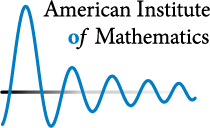# Arithmetic statistics over finite fields and function fields

January 27 to January 31, 2014

at the

American Institute of Mathematics, Palo Alto, California

organized by

Alina Bucur, Chantal David, and Jordan Ellenberg

This workshop, sponsored by AIM and the NSF, will be devoted to the study of statistical questions about objects of arithmetic geometry, especially algebraic varieties over function fields and finite fields. Some typical questions in arithmetic statistics are the following. What is the probability that a random integer is square free, or is prime? How many number fields of degree $d$ are there with discriminant of absolute value at most $X$? What does the class group of a random quadratic field look like? Many aspects of the subject are well-understood, but many more remain the subject of conjectures, by Cohen-Lenstra, Malle, Bhargava, Batyrev-Manin, and others.

In this workshop, we will concentrate on those questions with a special emphasis on what the statistics looks like when we start from the field $\mathbb{F}_{q}(t)$ of rational functions over a finite field (or, more generally, the function field of a curve over $\mathbb{F}_{q}$) instead of the field $\mathbb{Q}$ of rational numbers. Then the questions about number fields become questions about covers of the projective line $\mathbb{P}^1$, the questions about class groups become questions about groups of rational points on Jacobians, and so on. The main topics for the workshop are:

• formulate a probabilistic model for the large genus limit and fixed finite field of definition $\mathbb{F}_{q}$
• understand how the distribution of points on finite curves and zeta zeroes changes with the arithmetic invariant used
• understand which statistics for families over finite fields can be used to actually prove results about statistics over number fields, as in the work of Entin, Roditty-Gershon, and Rudnick
The analogy between function fields and number fields has been a rich source of insights throughout the modern history of number theory. In this setting, three active directions of number theory and algebraic geometry meet. The first direction is techniques imported from classical analytic number theory, which often must be modified and improved in the function field setting (sieve methods, Hardy-Littlewood method, etc.) The second direction is the theory of random matrices, which in this setting involves not only the much- studied properties of random complex matrices but those of random $l$-adic matrices as well. Finally, following Katz-Sarnak, arithmetic statistical questions over function fields can often be phrased as problems of counting $\mathbb{F}_{q}$-rational points on moduli schemes defined over $\mathbb{Z}$: such problems are deeply connected with the algebraic geometry and algebraic topology of the manifolds formed by the complex points on these moduli spaces.

Methods from a number of areas have been brought to bear on the subject, ranging from the Grothendieck ring of motives to the circle method of classical analytic number theory to ́etale cohomology and random matrix theory. An appealing example is presented by the work of Yu-Ru Liu and Trevor Wooley, and very recently of Siu-Lun Alan Lee and Thibaut Pugin, about Waring's problem and Birch's theorem over function fields, which draws connections between the circle method over function fields and geometric properties of spaces of rational curves on complete intersections. New methods have been developed, like the sieve developed by Bjorn Poonen to study the equivalent of the Bertini theorem in finite fields.

Finally, in the light of the spectacular recent work of Entin, Roditty-Gershon, and Rudnick it seems that statistics for families over finite fields can be used to actually prove results about statistics over number fields in some cases, and not only predict those statistics.

The workshop will differ from typical conferences in some regards. Participants will be invited to suggest open problems and questions before the workshop begins, and these will be posted on the workshop website. These include specific problems on which there is hope of making some progress during the workshop, as well as more ambitious problems which may influence the future activity of the field. Lectures at the workshop will be focused on familiarizing the participants with the background material leading up to specific problems, and the schedule will include discussion and parallel working sessions.

The deadline to apply for support to participate in this workshop has passed.